1800-1023-196

+91-120-4616500

# Electrochemical Series

## Electrochemical Series

(1) The standard reduction potentials of a large number of electrodes have been measured using standard hydrogen electrode as the reference electrode. These various electrodes can be arranged in increasing or decreasing order of their reduction potentials. The arrangement of elements in order of increasing reduction potential values is called electrochemical series. It is also called activity series, of some typical electrodes.

(2) Characteristics of Electrochemical series

(i) The negative sign of standard reduction potential indicates that an electrode when joined with SHE acts as anode and oxidation occurs on this electrode. For example, standard reduction potential of zinc is –0.76 volt, When zinc electrode is joined with SHE, it acts as anode (–ve electrode) i.e., oxidation occurs on this electrode. Similarly, the +ve sign of standard reduction potential indicates that the electrode when joined with SHE acts as cathode and reduction occurs on this electrode.

(ii) The substances, which are stronger reducing agents than hydrogen are placed above hydrogen in the series and have negative values of standard reduction potentials. All those substances which have positive values of reduction potentials and placed below hydrogen in the series are weaker reducing agents than hydrogen.

(iii) The substances, which are stronger oxidising agents than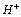ion are placed below hydrogen in the series.

(iv) The metals on the top (having high negative value of standard reduction potentials) have the tendency to lose electrons readily. These are active metals. The activity of metals decreases from top to bottom. The non-metals on the bottom (having high positive values of standard reduction potentials) have the tendency to accept electrons readily. These are active non-metals. The activity of non-metals increases from top to bottom.

Table : Standard reduction electrode potentials at 298K

Element Electrode Reaction (Reduction) Standard Electrode Reduction potential E0, volt
Li Li++ e = Li –3.05
##### K
K++ e = K –2.925
Ba Ba+++ 2e = Ba –2.90
Sr Sr+++ 2e = Sr –2.89
Ca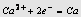–2.87
Na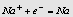–2.714
Mg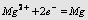–2.37
Al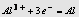–1.66
Mn Mn+++ 2e = Mn –1.18
Zn Zn2+ +2e=Zn –0.7628
Cr Cr3++3 e = Cr –0.74
Fe Fe2++ 2e = Fe –0.44
Cd Cd2++2e = Cd –0.403
Co Co+++ 2e = Co –0.27
Ni Ni2++2e = Ni –0.25
Sn Sn2++2e = Sn –0.14
##### Pb
Pb+++ 2e = Pb –0.12
H2 2H++2e = H2 0.00
Cu Cu2++ 2e = Cu +0.337
I2 I2+2e = 2I +0.535
Hg Hg2++2e = Hg +0.885
Ag Ag++ e = Ag +0.799
Br2 Br2+2e = 2Br +1.08
Pt Pt+++ 2e = Pt +1.20
Cl2 Cl2+2e = 2Cl +1.36
Au Au 3++3e = Au +1.50
F2 F2+2e= 2F +2.87

## NEET & AIIMS Exam Sample Papers

 AIIMS SAMPLE PAPERS View More NEET SAMPLE PAPERS View More